# ML Aggarwal Solutions for Class 7 Maths Chapter 12 Congruence of Triangles

ML Aggarwal Solutions for Class 7 Maths Chapter 12 Congruence of Triangles are given for easy understanding of the key concepts present in this chapter. The solutions are designed in such a manner that the students will be able to grasp the concepts easily. This chapter mainly deals with problems based on the Congruence of Triangles. For a better understanding of the concepts, students can solve the exercise problems using the ML Aggarwal Solutions. All the solutions are designed by subject experts at BYJU’S, according to the latest ICSE guidelines. Using this resource on a regular basis, students can achieve high scores in their examinations.

Chapter 12 Congruence of Triangles gives answers to questions related to all the topics covered in this chapter. Further, students can refer to ML Aggarwal Class 7 Solutions PDF, which can be downloaded for free from the links given below.

## Download the PDF of ML Aggarwal Solutions for Class 7 Maths Chapter 12 Congruence of Triangles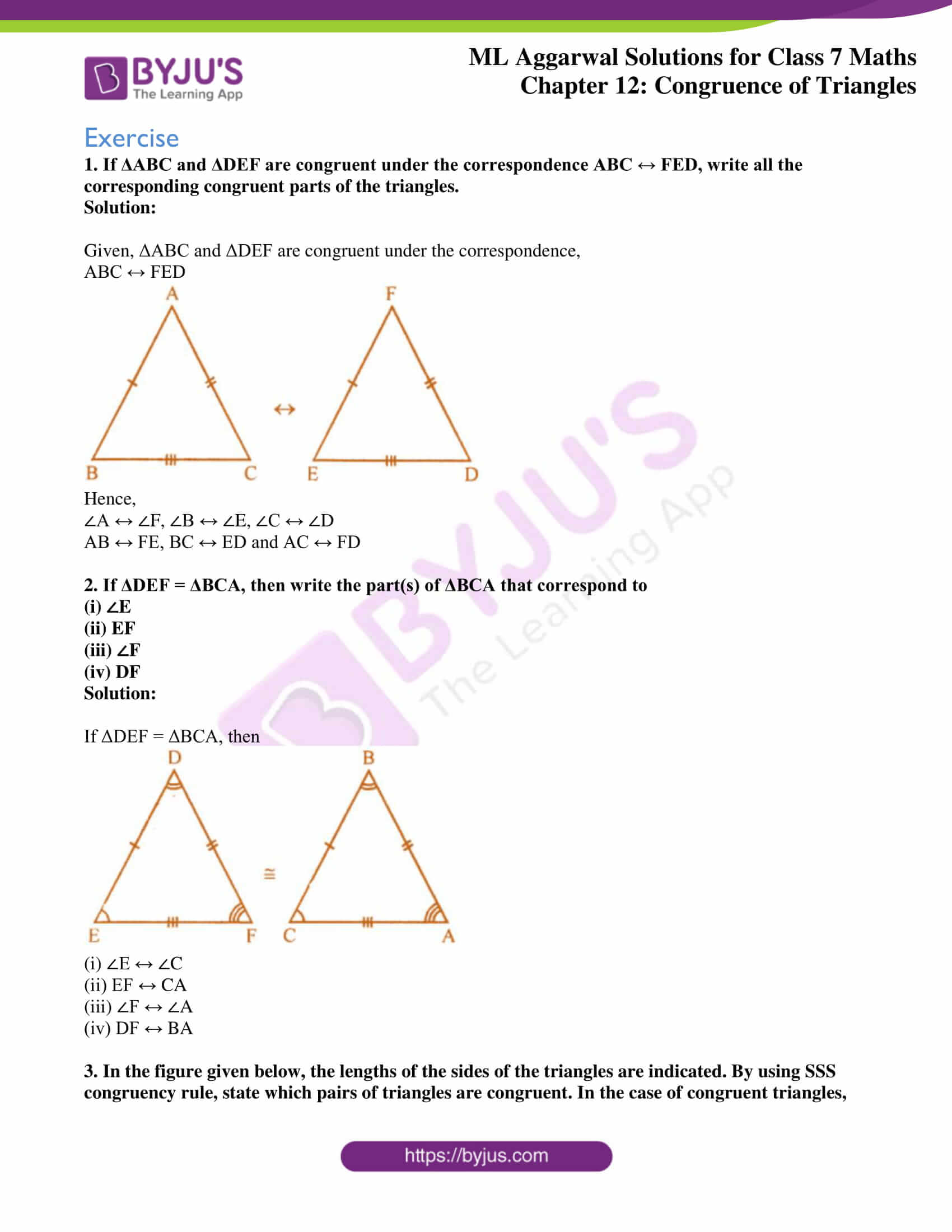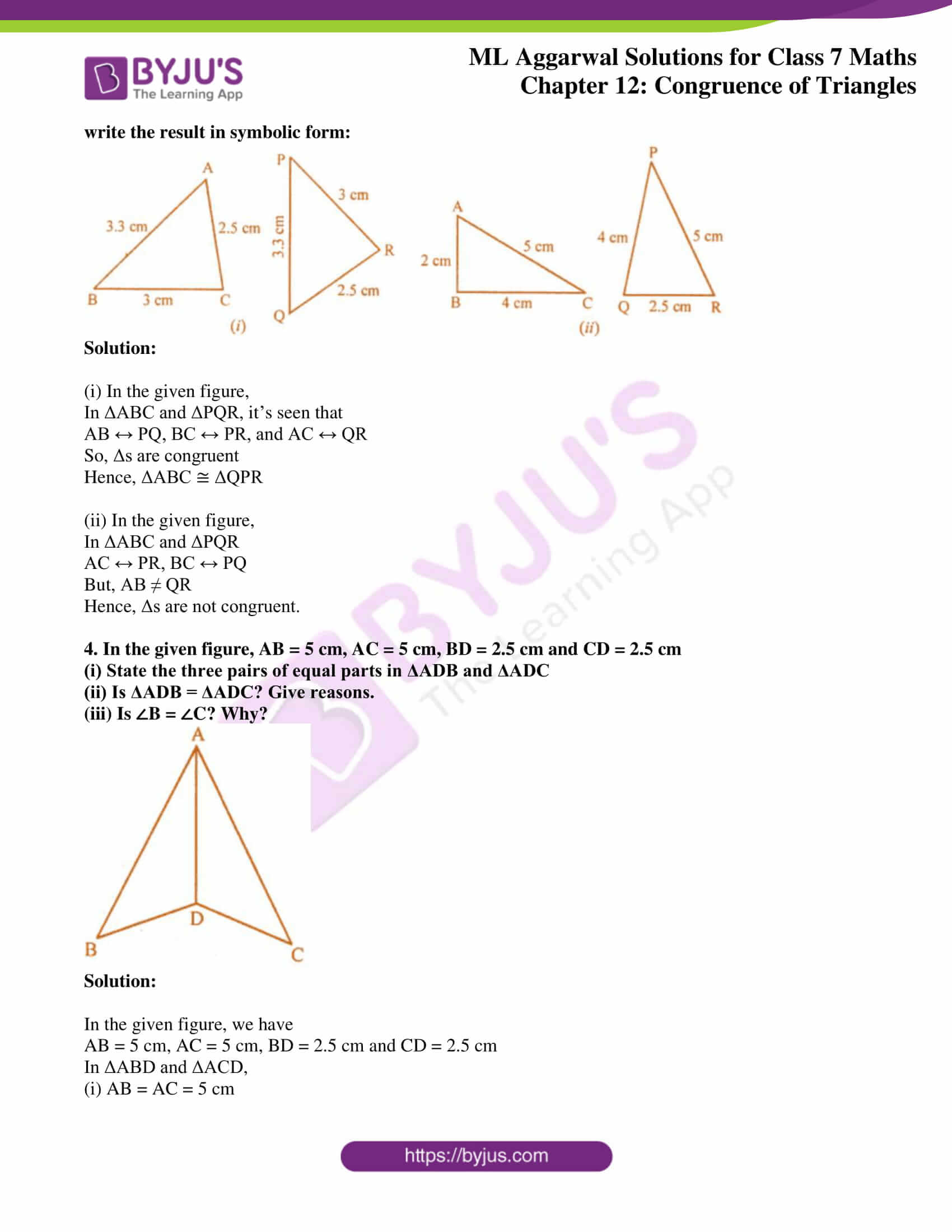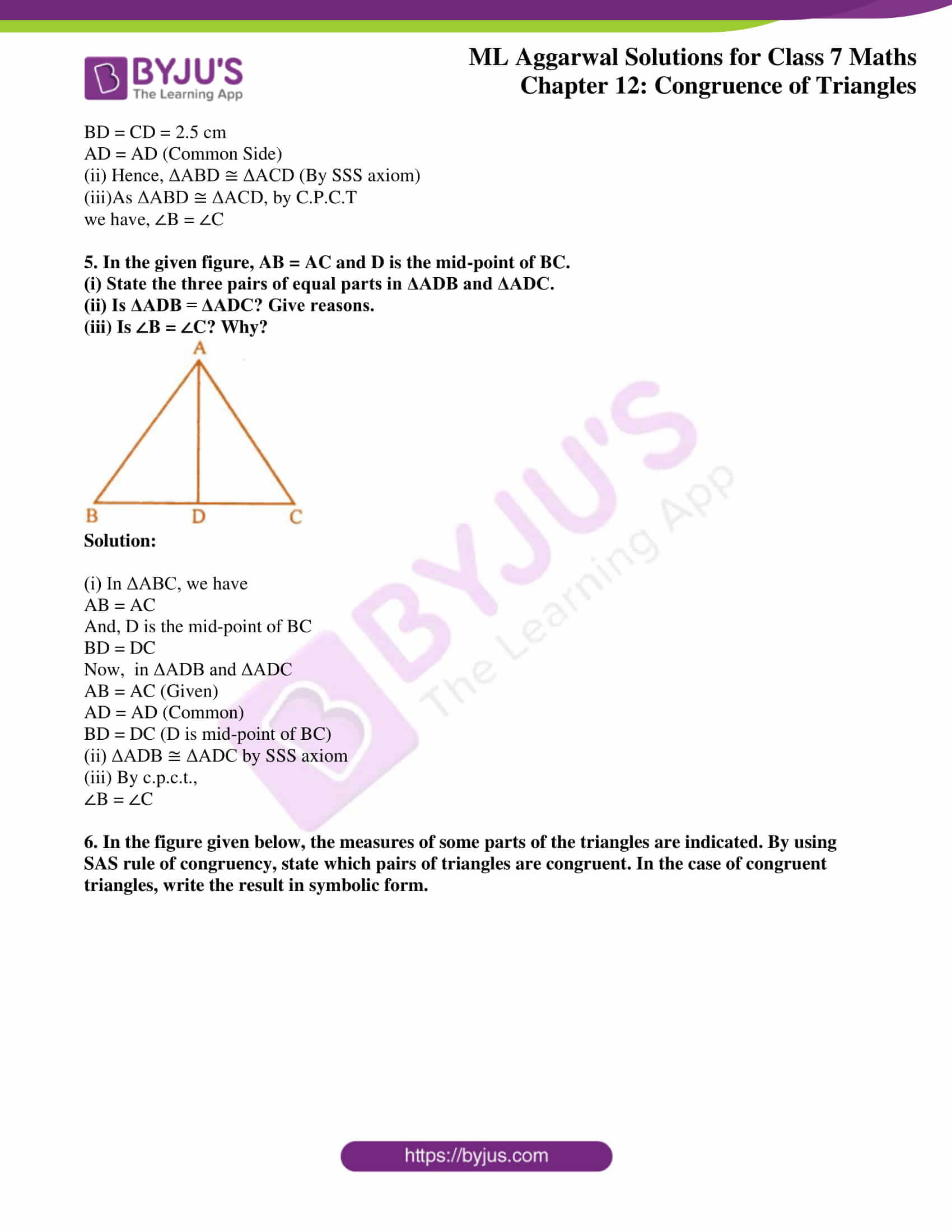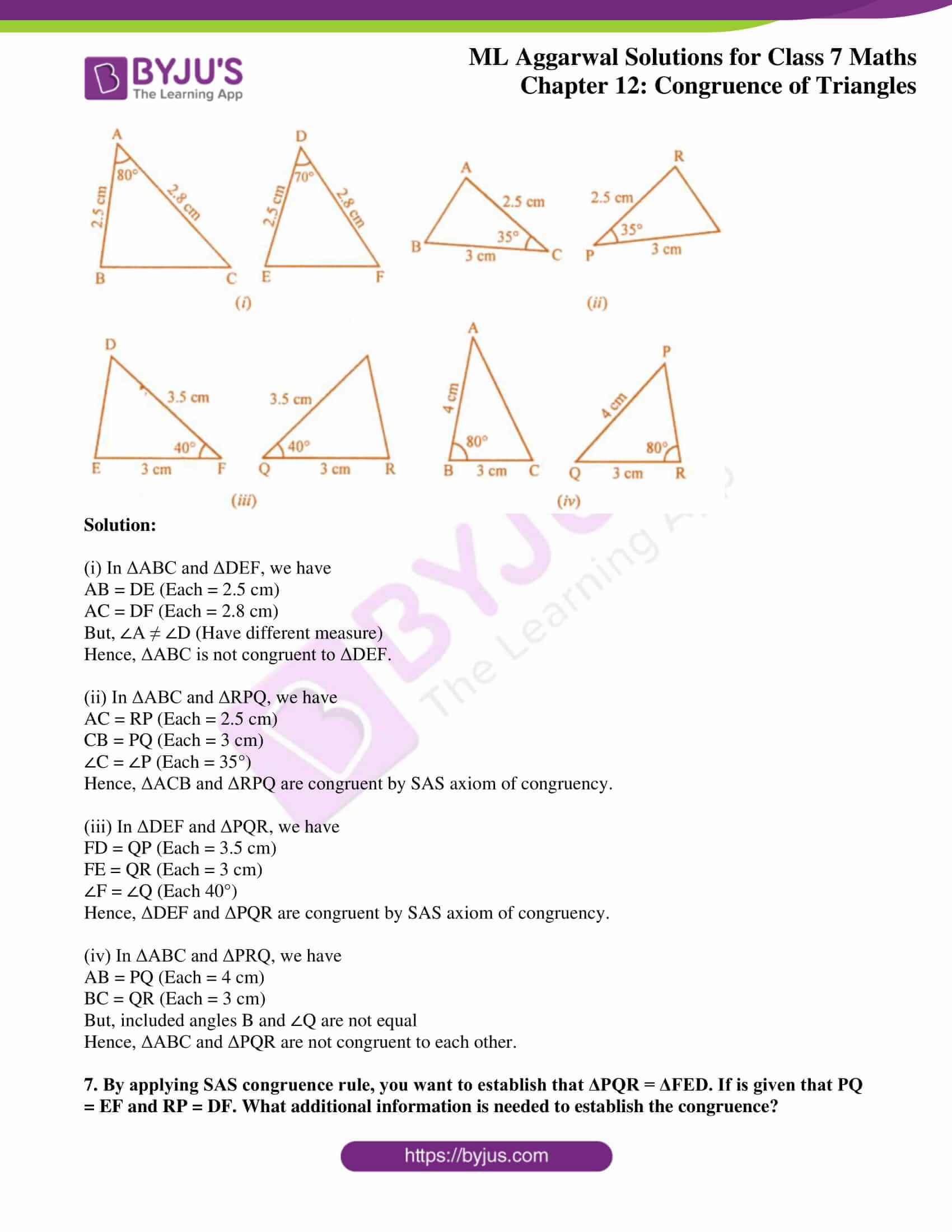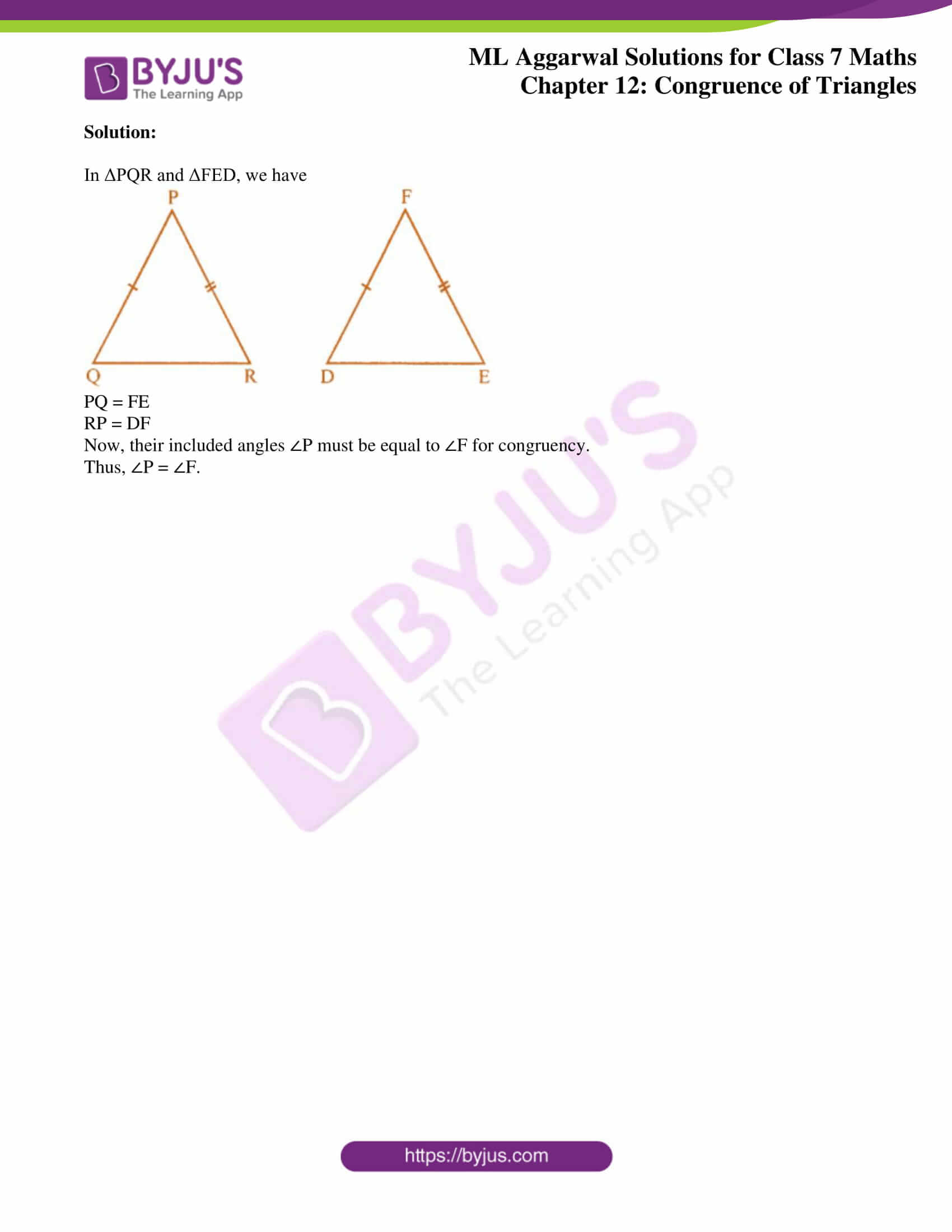### Access answers to ML Aggarwal Solutions for Class 7 Maths Chapter 12 Congruence of Triangles

Exercise
1. If ΔABC and ΔDEF are congruent under the correspondence ABC ↔ FED, write all the corresponding congruent parts of the triangles.
Solution:

Given, ΔABC and ΔDEF are congruent under the correspondence,

ABC ↔ FED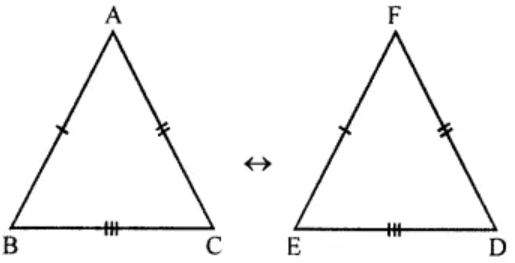Hence,

∠A ↔ ∠F, ∠B ↔ ∠E, ∠C ↔ ∠D

AB ↔ FE, BC ↔ ED and AC ↔ FD

2. If ΔDEF = ΔBCA, then write the part(s) of ΔBCA that correspond to
(i) ∠E
(ii) EF
(iii) ∠F
(iv) DF
Solution:

If ΔDEF = ΔBCA, then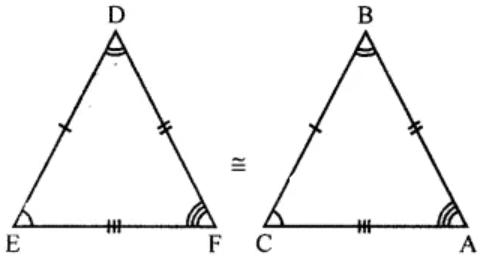(i) ∠E ↔ ∠C

(ii) EF ↔ CA

(iii) ∠F ↔ ∠A

(iv) DF ↔ BA

3. In the figure given below, the lengths of the sides of the triangles are indicated. By using SSS congruency rule, state which pairs of triangles are congruent. In the case of congruent triangles, write the result in symbolic form: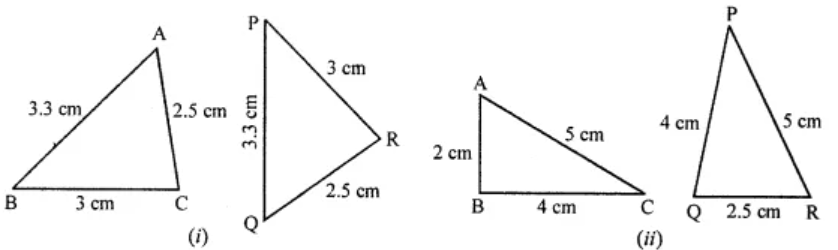Solution:

(i) In the given figure,

In ΔABC and ΔPQR, it’s seen that

AB ↔ PQ, BC ↔ PR, and AC ↔ QR

So, Δs are congruent

Hence, ΔABC ≅ ΔQPR

(ii) In the given figure,

In ΔABC and ΔPQR

AC ↔ PR, BC ↔ PQ

But, AB ≠ QR

Hence, Δs are not congruent.

4. In the given figure, AB = 5 cm, AC = 5 cm, BD = 2.5 cm and CD = 2.5 cm
(i) State the three pairs of equal parts in ΔADB and ΔADC
(iii) Is ∠B = ∠C? Why?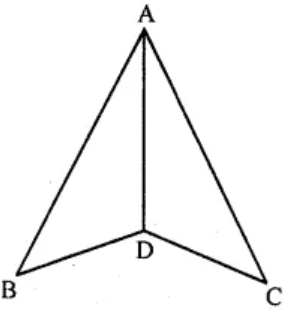Solution:

In the given figure, we have

AB = 5 cm, AC = 5 cm, BD = 2.5 cm and CD = 2.5 cm

In ΔABD and ΔACD,

(i) AB = AC = 5 cm

BD = CD = 2.5 cm

(ii) Hence, ΔABD ≅ ΔACD (By SSS axiom)

(iii)As ΔABD ≅ ΔACD, by C.P.C.T

we have, ∠B = ∠C

5. In the given figure, AB = AC and D is the mid-point of BC.
(i) State the three pairs of equal parts in ΔADB and ΔADC.
(iii) Is ∠B = ∠C? Why?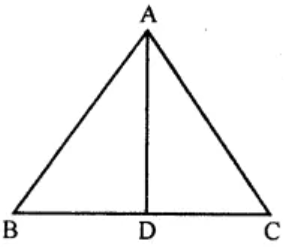Solution:

(i) In ΔABC, we have

AB = AC

And, D is the mid-point of BC

BD = DC

AB = AC (Given)

BD = DC (D is mid-point of BC)

(iii) By c.p.c.t.,

∠B = ∠C

6. In the figure given below, the measures of some parts of the triangles are indicated. By using SAS rule of congruency, state which pairs of triangles are congruent. In the case of congruent triangles, write the result in symbolic form.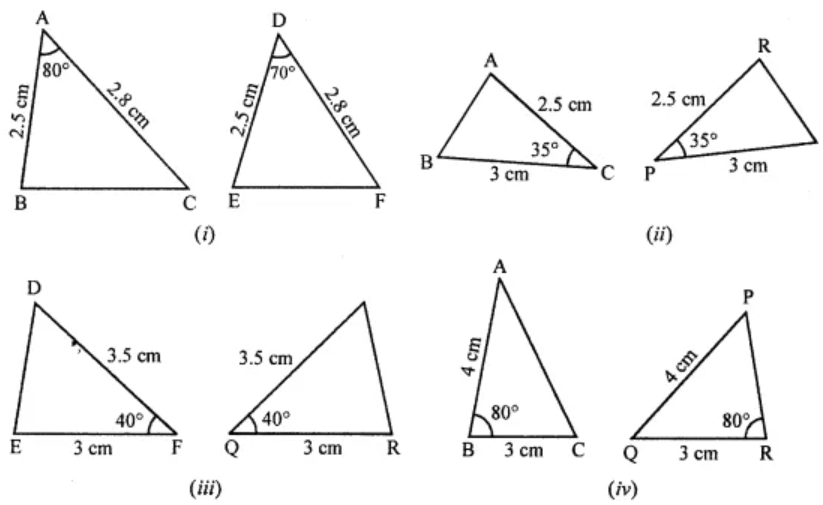Solution:

(i) In ΔABC and ΔDEF, we have

AB = DE (Each = 2.5 cm)

AC = DF (Each = 2.8 cm)

But, ∠A ≠ ∠D (Have different measure)

Hence, ΔABC is not congruent to ΔDEF.

(ii) In ΔABC and ΔRPQ, we have

AC = RP (Each = 2.5 cm)

CB = PQ (Each = 3 cm)

∠C = ∠P (Each = 35°)

Hence,
ΔACB and ΔRPQ are congruent by SAS axiom of congruency.

(iii) In ΔDEF and ΔPQR, we have

FD = QP (Each = 3.5 cm)

FE = QR (Each = 3 cm)

∠F = ∠Q (Each 40°)

Hence, ΔDEF and ΔPQR are congruent by SAS axiom of congruency.

(iv) In ΔABC and ΔPRQ, we have

AB = PQ (Each = 4 cm)

BC = QR (Each = 3 cm)

But, included angles B and ∠Q are not equal

Hence, ΔABC and ΔPQR are not congruent to each other.

7. By applying SAS congruence rule, you want to establish that ΔPQR = ΔFED. If is given that PQ = EF and RP = DF. What additional information is needed to establish the congruence?
Solution:

In ΔPQR and ΔFED, we havePQ = FE

RP = DF

Now, their included angles ∠P must be equal to ∠F for congruency.

Thus, ∠P = ∠F.